To do source reconstruction of MEG signals we need to solve the inverse problem—i.e., find the sources that generate the magnetic field patterns that we measure. This inverse problem has infinite solutions. To be able to estimate the sources of the magnetic signals we need constraints on the possible solutions. Lucky enough, we know that the origin of MEG signals is not any random electric currents, but currents in the brain—more precise the pyramidal cells in neocortex. We use this information to constrain the solutions to the inverse problem to a set of pre-specified locations. We assume that the activity we measure comes from the brain and thus limit our possible sources to be within the brain.

We do this by constraining the inverse solution based on the anatomy of the brain and head, information about the volume containing the electric fields (the volume conduction model), and information about the location of the head relative to the MEG sensors.

To do source reconstruction of MEG and EEG signals, we need a model of the head and how it conducts electrical currents. We obtain a model of the head and the brain from a structural magnetic resonance image (MRI). There are three basic ingredients for source reconstruction:

1. MEG data.
2. A structural MRI.

Usually, we will want individual MRI for each participant to accommodate individual differences in head geometry and shape of the brain, but in some cases, it can be sufficient to use a template brain.

In this tutorial, we have an MRI for the subject. The raw dicom files are located in a separate folder. The information about the relative location of sensors to the head is contained in the raw MEG data. In addition, there is a number of head points that we “drew” on the subject’s head. We will use these points to align MEG and MRI data.

In this tutorial, we will create a volume conductor model of the subject’s head.

### Set up general paths

Make sure we have FieldTrip in our Matlab path and specify data paths. Change these to appropriate paths for your operating system and setup.

``````restoredefaultpath
% CHANGE THE FieldTrip PATH ACCORDING TO YOUR SYSTEM
ft_defaults

data_path = '/home/share/workshops_MEG_Nord/mikkel_and_lau/data/';
meg_path = fullfile(data_path, 'sub-01/ses-meg/meg');
dcm_path = fullfile(data_path, 'sub-01/ses-mri/anat');
mri_path = fullfile(data_path, 'sub-01/ses-mri/');``````

Dicom is a standard file format for MRI. If you look in the dicom directory, you see several files. Each dicom file contains a slice of the MRI volume. We read the dicom files with the function ft_read_mri. To read the dicoms into a single volume, it is enough to provide the filename of any of the dicom in the sequence, ft_read_mri will recognize the rest of the files and read all of them (assuming they are in the same directory).

``````dicom_name = '00000001.dcm';
dicom_path = fullfile(dcm_path, dicom_name);

Take a look at the newly created mri structure.

You can plot the MRI volume with the function ft_sourceplot . Be patient as it might take some time to generate the plots due to the large size of the data. (if it takes too long to plot the volumes, just skip the plotting that is only for inspection purposes).

``````figure
ft_sourceplot([], mri);``````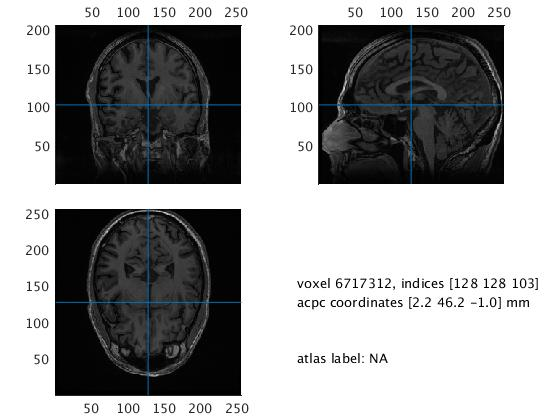Do not mind that the function is called ft_sourceplot, it is not yet the source space we are looking at.

## Co-register MEG and MRI data

To align the MRI image to the head points in the MEG data, the first step is to make sure they are in the same coordinate system. As a default, dicom files are in the native coordinate system of the MRI scanner, whereas the MEG data are in a coordinate system defined by the MEG scanner. These coordinate systems are defined in different ways.

There a different ways to define head coordinate systems. Different devises use different default coordinate systems. You can read more about how the coordinate systems are defined here: http://www.fieldtriptoolbox.org/faq/how_are_the_different_head_and_mri_coordinate_systems_defined

The tutorial dataset was collected on an Electra Neuromag MEG scanner and use the Neuromag coordinate system. The Neuromag coordinate system is defined frim external landmarks – i.e., the fiducials, we defined as the first step when we digitized the subject. The coordinate system is defined like this:

• X-axis from the origin towards the RPA point (Right is “up” on X-axis)
• Y-axis from the origin towards the nasion (posterior is “up” on Y-axis)
• Z-axis from the origin upwards orthogonal to the XY-plane (superior is “up” on Z-axis)
• Origin: The intersection of the X-axis and Y-axis.

We transform everything into the Neuromag coordinate system. ,The coordinate system we use is the “native” system of the MEG data. What coordinate system we use is just a practical formality to co-register the MRI and MEG data. This procedure is the same for any coordinate system your raw MEG data might be defined by. Transformation to anatomical coordinates such as Talairach-Tournoux or MNI is a different transformation, usually done at another point in the processing.

### Indicate coordinate system

The first step is to define the axes of the coordinate system. We do this with the FieldTrip function ft_determine_coordsys. When you call ft_determine_coordsys a figure will pop up with the axes on top of the MRI.

``````%% determine coordinate system

mri_coordsys = ft_determine_coordsys(mri);
``````

A figure will pop up. Take a look at the direction of the axes.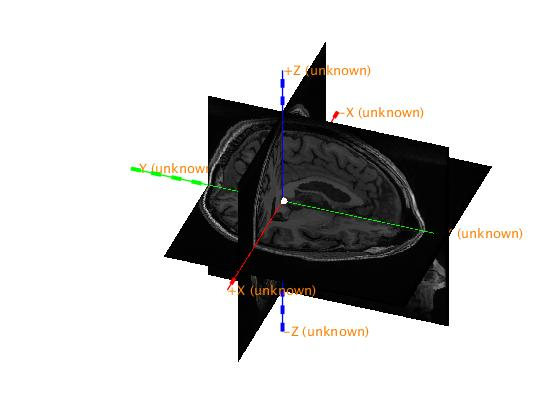From the figure, you have to specify the X, Y, and Z-axes. You can rotate the figure to find the directions of the axes, e.g. find the nose as a landmark.

In the Matlab terminal, you are asked if you want to change the anatomical landmarks. Press “y” and “Enter” to specify the direction of the axes. FieldTrip is then asking you to provide information about the direction of the axes.

>>What is the anatomical label for the positive X-axis [r, l, a, p, s, i]?

>>What is the anatomical label for the positive Y-axis [r, l, a, p, s, i]?

>>What is the anatomical label for the positive Z-axis [r, l, a, p, s, i]?

Press the corresponding letters that matches the positive direction of the three axes r(ight), l(eft), a(nterior), p(osterior), s(uperior), i(nferior).

When you have defined the axes, it is a good idea to save the MRI.

``save([mri_path 'mri_coordsys'], 'mri_coordsys');``

### Co-register MRI to Neuromag based on fiducials (nasion and left and right pre-auricular points)

Now we will co-register the MRI image to the head points. In FieldTrip this is done in a step-wise procedure.

First, we align the MRI to the fiducial points. To align MRI and fiducials, we need to specify the fiducials on the MRI. We do this with the FieldTrip function ft_volumerealign. This call will again plot the volume. You now have to mark the approximate location of the fiducial points NAS, LPA, and RPA.

``````%% Coregistration
cfg = [];
cfg.method   = 'interactive';
cfg.coordsys = 'neuromag';

mri_realigned_1 = ft_volumerealign(cfg, mri_coordsys);
``````

Click on the image until the crosshair is on a location corresponding to a fiducial point. You can also scroll through the volume with the arrow keys. Once the crosshair is in a location you think is (approximately) correct, press “n” for NAS, “l” for LPA, and “r” for RPA on your keyboard. The coordinates of the fiducials will be written in the MATLAB terminal. Press “f” on your keyboard to toggle fiducial visibility on the plot.

You can mark the fiducials multiple times until you are satisfied with the positions. Do it as precise as you can, but do not get upset if it is not perfect. Imprecision will be adjusted in the following steps.

Finally, you are asked to provide an extra control point that should have a positive value on the Z-axis. Click somewhere on the volume that you are sure has a positive Z-value (e.g., the top of the scalp) and press z. (This helps in figuring out whether you have correctly identified left and right)

Press q when you are done and close the figure.

At this point, you want to save the data again.

``save(fullfile(mri_path, 'mri_realigned_1'), 'mri_realigned_1');``

### Co-register with the extra digitization points (along the scalp and the face)

``````%% Read headpoints and sensor geometry

Plot the points

``ft_plot_headshape(headshape);``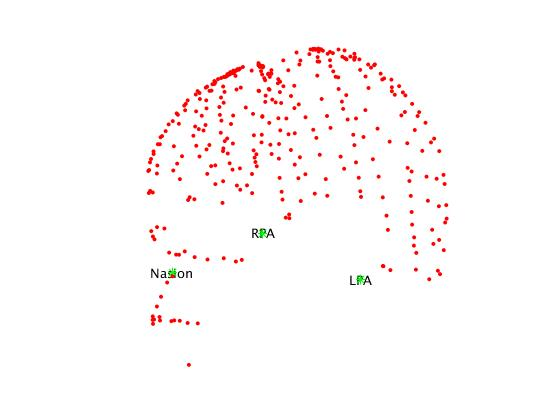The head points are in the Neuromag coordinate system together with the location of the head relative to the MEG sensors. Let us load the MEG sensor locations and inspect:

``````sens = ft_read_sens(fullfile(meg_path, headshape_file),'senstype','meg'); % Load MEG sensors

figure;
ft_plot_sens(sens);               % Plot MEG sensors
``````

We then use ft_volumerealign again, but this time we specify that we want to use an automatic procedure called “iterative closest point” (icp) to fit fiducials and head points.

``````%% Align MRI to headpoints
cfg = [];
cfg.coordsys            = 'neuromag';

mri_realigned_2 = ft_volumerealign(cfg, mri_realigned_1);
``````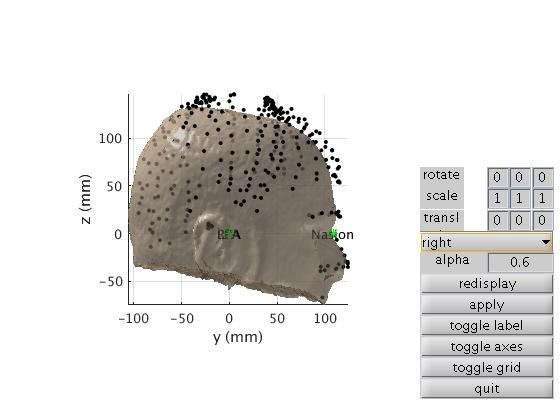### Check co-registration

This step is really just used to check whether the coregistration went okay.

``````% Checking co-registration
cfg = [];
cfg.coordsys            = 'neuromag';
cfg.headshape.icp       = 'no';        %Do not fit point again

mri_realigned_3 = ft_volumerealign(cfg, mri_realigned_2);``````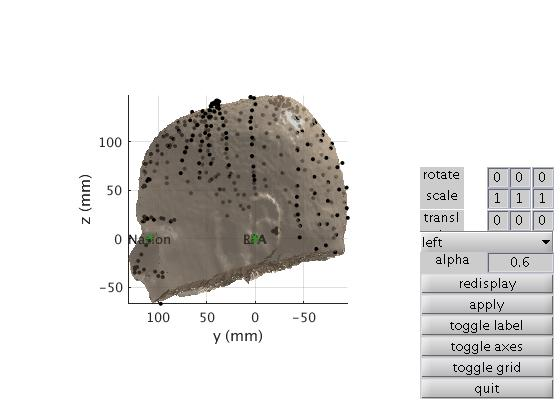Save the final realigned MRI.

``````% Save volume
save([mri_path 'mri_realigned_3'], 'mri_realigned_3');
``````

### Reslice MRI data

The next step is to reslice the MRI on to a 1x1x1 mm cubic grid aligned with the coordinate axes.

``````## Reslice MRI data
cfg = [];
cfg.resolution = 1;

mri_resliced = ft_volumereslice(cfg, mri_realigned_3);``````

To avoid potential errors due to coordinates systems being declared in different units, we make sure the MRI is defined in centimeters:

``````%% Convert unit
mri_resliced_cm = ft_convert_units(mri_resliced, 'cm');``````

## Segment data into brain, skull, and scalp

We will segment the volume into three compartments: One for the brain, one for the skull, and one for the scalp. This is done automatically with the function ft_volumesegment. All it needs is a configuration specifying which output segments we want.

``````cfg = [];
cfg.output = {'brain' 'skull' 'scalp'};

mri_segmented = ft_volumesegment(cfg, mri_resliced_cm);

save([mri_path 'mri_segmented'], 'mri_segmented');``````

Now it is a good idea to inspect that the segmentation went well.

``````cfg = [];
cfg.funparameter = 'brain';
ft_sourceplot(cfg, mri_segmented);

cfg.funparameter = 'skull';
ft_sourceplot(cfg, mri_segmented);

cfg.funparameter = 'scalp';
ft_sourceplot(cfg, mri_segmented);``````

Brain segment: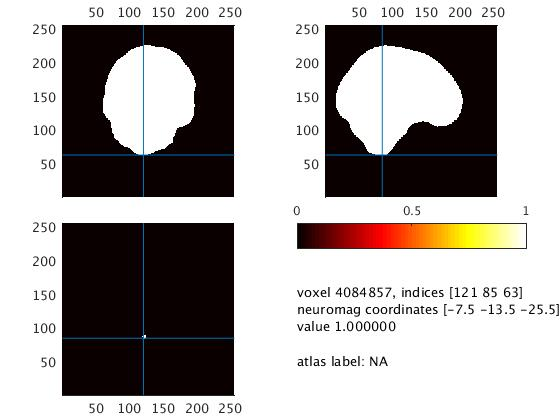Skull segment: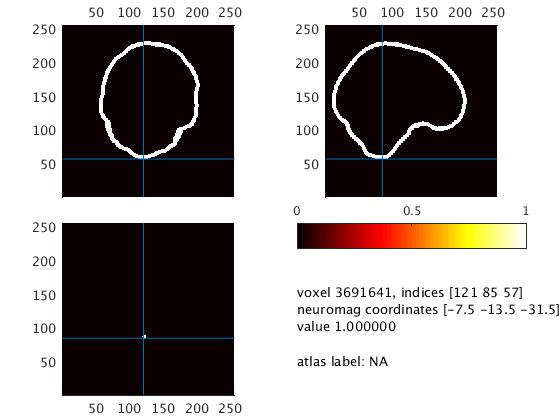Scalp segment: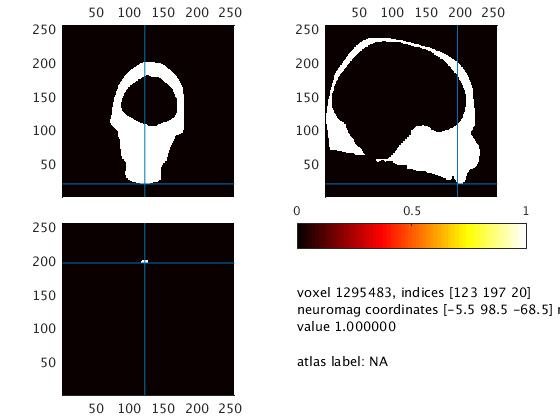## Construct mesh

We now define the border between the inner skull and the brain. For MEG we are (usually) only interested in the inside compartment for our volume conduction model. Note that if we would want to do the same for EEG, we need to model the different segments we created above in a multi-layer model.

We define a mesh from the border between the inner skull and the brain. We will call this mesh brain. To prepare the mesh, we use the FieldTrip function ft_prepare_mesh. We specify the number of vertices on the surface with the option cfg.numverticies.

``````%% Construct mesh
cfg = [];
cfg.method = 'projectmesh';
cfg.tissue = 'brain';
cfg.numvertices = 3000;

mesh_brain = ft_prepare_mesh(cfg, mri_segmented);``````

Plot the mesh to see if it looks fine:

``ft_plot_mesh(mesh_brain)``